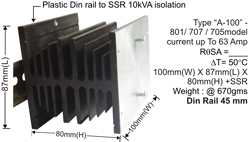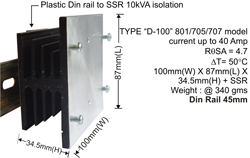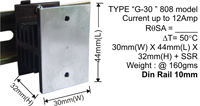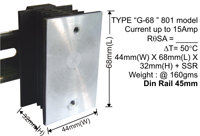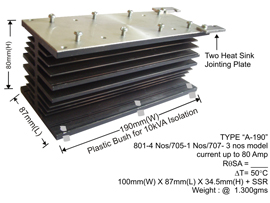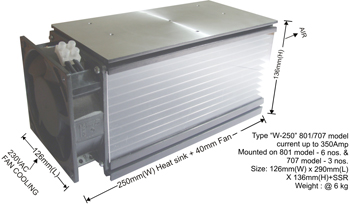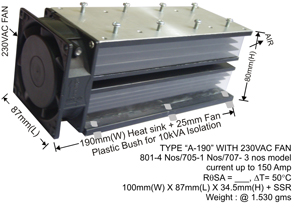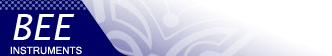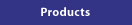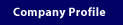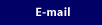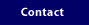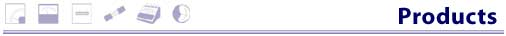Products >>>Relays >>> Heat Sink

Heat Sink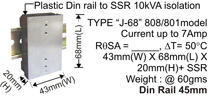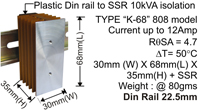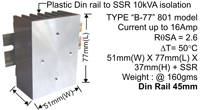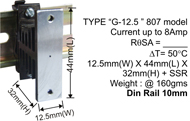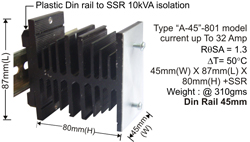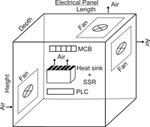• Heat Sink Fins should be in vertical position So that hot
air flow from bottom to top - self cooling
• Keep 20mm gap at top and bottom of heat sink.
• Apply Heat Sink compound between SSR and heat sink
• The screw should tight properly so no air gap between
SSR and Heat Sink

• 1800 square mm of total exposed aluminum is sufficient to dissipated one watt of heat generated.

Thernal Calculations
 Tj - TA = P(RθJC + RθSA) Tj = Junction Temperature (°C) 125°C TA =Ambient Temperature (°C) P =Power dissipation (watts)Voltage drop x load current RθJC =Thermal resistance junction to case °CW RθCS =Thermal resistance of Heat sink compound (o.2°C/WTYP) RθSA Thermal resistance of external heat sink(°C/W) it depend upon length, width, expose aluminum (0.5 to 5)
If SSR current capacity high and SSR use for lower capacity of load current than heat sink size will reduced Example:
• 50Amp SSR used for 15Amp Load Current than “B” Type of Heat sink.
• 50Amp SSR used for 25Amp load current then “C” type of Heat Sink.
•